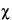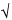GLOSSARY
Glossary of Symbols
 A absorbance for computing % reduction in bacterial growth from turbidimetric readings. b slope of the straight line relating response (y) to log-dose (x) [Equations 2b, 4, 5, 6]. c constant for computing M¢ with Equations 8 and 10. c¢ constant for computing L with Equations 26 and 29. ci constant for computing M¢ when doses are spaced as in Table 8. c ¢ i2 constant for computing L when doses are spaced as in Table 8. C term measuring precision of the slope in a confidence interval [Equations 27, 28, 35, 36].2 statistical constant for testing significance of a discrepancy [Table 9].M22 testing the disagreement between different estimates of log-potency [Equations 39, 40]. eb ei from row b in Tables 6 to 8. eb¢ i multiple of S(x – x)2 [Table 5; Equation 6]. ei sum of squares of the factorial coefficients in each row of Tables 6 to 8. eq ei from row q in Tables 6 to 8. f number of responses at each dosage level of a preparation; number of replicates or sets. fS number of observations on the Standard. fU number of observations on the Unknown. F1 to F3 observed variance ratio with 1 to 3 degrees of freedom in numerator [Table 9]. G1, G2, and G3 relative gap in test for outlier [Table 1]. h number of Unknowns in a multiple assay. h¢ number of preparations in a multiple assay, including the Standard and h Unknowns; i.e., h¢ = h + 1. i interval in logarithms between successive log-doses, the same for both Standard and Unknown. k number of estimated log-potencies in an average [Equation 24]; number of treatments or doses [Table 4; Equations 1, 13, 15, 16]; number of ranges or groups in a series [Table 2]; number of rows, columns, and doses in a single Latin square [Equations 1a, 16a]. L length of the confidence interval in logarithms [Equations 24, 26, 29, 38], or in terms of a proportion of the relative potency of the dilutions compared [Equations 31, 33]. Lc length of a combined confidence interval [Equations 42, 43]. Lc¢ length of confidence interval for a semi-weighted mean M [Equation 48]. LD50 lethal dose killing an expected 50% of the animals under test [Equation 2c]. M log-potency [Equation 2]. M¢ log-potency of an Unknown, relative to its assumed potency. M mean log-potency. n degrees of freedom in an estimated variance s2 or in the statistic t or2. n¢ number of Latin squares with rows in common [Equations 1a, 16a]. N number; e.g., of observations in a gap test [Table 1], or of responses y in an assay [Equation 16]. P probability of observing a given result, or of the tabular value of a statistic, usually P = 0.05 or 0.95 for confidence intervals [Tables 1, 2, 9]. P* potency, P* = antilog M or computed directly. R ratio of a given dose of the Standard to the corresponding dose of the Unknown, or assumed potency of the Unknown [Equations 2, 30, 33]. R* ratio of largest of k ranges in a series to their sum [Table 2]. s =s2 standard deviation of a response unit, also of a single estimated log-potency in a direct assay [Equation 24]. s2 error variance of a response unit. Si a log-dose of Standard [Tables 6, 7]. S “the sum of.” t Student's t for n degrees of freedom and probability P = 0.05 [Table 9]. T total of the responses y in an assay [Equation 16]. T ¢ incomplete total for an assay in randomized sets with one missing observation [Equation 1]. T1 S(y) for the animals injected with the Standard on the first day [Equations 18, 36]. T2 S(y) for the animals injected with the Standard on the second day [Equations 18, 36]. Ta Ti for the difference in the responses to the Standard and to the Unknown [Tables 6 to 8]. Tab Ti for testing the difference in slope between Standard and Unknown [Tables 6 to 8]. Taq Ti for testing opposed curvature in the curves for Standard and Unknown [Tables 6 to 8]. Tb Ti for the combined slope of the dosage-response curves for Standard and Unknown [Tables 6 to 8]. Tb¢ S(x1Tt) or S(x1y) for computing the slope of the log-dose response curve [Equations 10, 23, 28]. Ti sum of products of Tt multiplied by the corresponding factorial coefficients in each row of Tables 6 to 8. Tq Ti for testing similar curvature in the curves for Standard and Unknown [Tables 6 to 8]. Tr row or set total in an assay in randomized sets [Equation 16]. Tr¢ incomplete total for the randomized set with a missing observation in Equation 1. Tt total of f responses y for a given dose of a preparation [Tables 6 to 8; Equations 6, 13, 14, 16]. Tt¢ incomplete total for the treatment with a missing observation in Equation 1. Ui a log-dose of Unknown [Tables 6 to 8]. v variance for heterogeneity between assays [Equation 45]. V = 1/w variance of an individual M [Equations 44 to 47]. w weight assigned to the M for an individual assay [Equation 38], or to a probit for computing an LD50 [Equations 2a, 2b]. w¢ semi-weight of each M in a series of assays [Equations 47, 48]. x a log-dose of drug in a bioassay [Equation 5]; also the difference between two log-threshold doses in the same animal [Equation 12]. x* coefficients for computing the lowest and highest expected responses YL and YH in a log-dose response curve [Table 4; Equation 3]. x1 a factorial coefficient that is a multiple of (x – x) for computing the slope of a straight line [Table 5; Equation 6]. x mean log-dose [Equation 5]. xS mean log-dose for Standard [Equation 9]. xU mean log-dose for Unknown [Equation 9]. X log-potency from a unit response, as interpolated from a standard curve [Equations 7a, 7b, 19]. XM confidence limits for an estimated log-potency M [Equations 25, 30]. XP* confidence limits for a directly estimated potency P* (see Digitalis assay) [Equation 33]. y an observed individual response to a dose of drug in the units used in computing potency and the error variance [Equations 13 to 16]; a unit difference between paired responses in 2-dose assays [Equations 17, 18]. y1 . . . yN observed responses listed in order of magnitude, for computing G1, G2, or G3 in Table 1. y¢ replacement for a missing value [Equation 1]. y mean response in a set or assay [Equation 5]. yt mean response to a given treatment [Equations 3, 6]. Y a response predicted from a dosage-response relationship,often with qualifying subscripts [Equations 3 to 5]. z threshold dose determined directly by titration (see Digitalis assay) [Equation 11]. z mean threshold dose in a set (see Digitalis assay) [Equations 31, 32, 33].